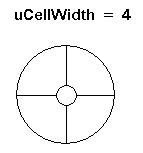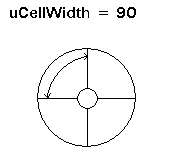←Select platform
In This Topic ▼

Summary
Initializes a new PixelateCommand class object with explicit parameters.
Syntax
C#
C++/CLI
Python
````public PixelateCommand( `
`   int cellWidth, `
`   int cellHeight, `
`   int opacity, `
`   LeadPoint centerPoint, `
`   PixelateCommandFlags flags `
`) ````
````public: `
`PixelateCommand(  `
`   int cellWidth, `
`   int cellHeight, `
`   int opacity, `
`   LeadPoint centerPoint, `
`   PixelateCommandFlags flags `
`) ````
``__init__(self,cellWidth,cellHeight,opacity,centerPoint,flags) # Overloaded constructor ``

#### Parameters

cellWidth
Value that represents the width of a rectangular cell, the number of rectangular cells present across the width of the image, the number of cells around the center point of a circular cell, or the length in degrees of each cell around the center point of a circular cell, based on the flags.

 If these flags are set: Then, the CellWidth property (or the cellWidth parameter) contains: And the range of possible values is: PixelateCommandFlags.Radial | PixelateCommandFlags.WidthFrequency the number of cells around the center point. That is, the circle around the center point is divided into equal parts, based on the value in the CellWidth property (or the cellWidth parameter if you are using the Constructor) as shown below:1 to 360. The circle can be divided into anywhere from 1 to 360 equal parts. PixelateCommandFlags.Radial | PixelateCommandFlags.WidthPeriod the size, in degrees of the cells around the center point That is, the circle around the center point is divided into cells based on the number of degrees set in the CellWidth property (or the cellWidth parameter if you are using the Constructor), as shown below:1 to 360. The circle can be divided into cells of 1 degree to 360 degrees. PixelateCommandFlags.Rectangle | PixelateCommandFlags.WidthFrequency the number of cells present across the width of the image. 1 to the image width, if there is no region. If there is a region, then the range of values is just the region width. PixelateCommandFlags.Rectangle | PixelateCommandFlags.WidthPeriod | the width of each rectangular cell, in pixels. 1 to the image width, if there is no region. If there is a region, then the range of values is just the region width.

cellHeight
Value that represents the height of a rectangular cell, the number of rectangular cells present across the height of the image, the number of cells along the radius of a circular cell, or the length of each cell along the radius of a circular cell, based on the flags.

 If the following flag is set: Then, the CellHeight property (or the cellHeight parameter if you are using the Constructor) contains: And the range of possible values is: PixelateCommandFlags.Radial | PixelateCommandFlags.HeightFrequency the number of cells present along the radius of the circular cell. 1 to the diagonal of the image, if there is no region. If there is a region, then the range of values is just the diagonal of the region rectangle. PixelateCommandFlags.Radial | PixelateCommandFlags.HeightPeriod the radial length of each circular cell, in pixels. 1 to the diagonal of the image, if there is no region. If there is a region, then the range of values is just the diagonal of the region rectangle. PixelateCommandFlags.Rectangle | PixelateCommandFlags.HeightFrequency the number of cells present across the height of the image. 1 to the image height, if there is no region. If there is a region, then the range of values is just the region height. PixelateCommandFlags.Rectangle | PixelateCommandFlags.HeightPeriod | the height of each rectangular cell, in pixels. 1 to the image height, if there is no region. If there is a region, then the range of values is just the region height.

opacity
Value that represents how transparent the cells are, compared to the original pixels. This is a percentage. Possible values are 0 - 100. (0 is total opacity and 100 is total transparency.)

centerPoint
Represents the center of revolution when circular cells are used. This parameter will be ignored if the PixelateCommandFlags.Rectangle flag is set.

flags
Flags that indicate the values used to fill the cells, the shape of the cells, and what type of data is in the CellWidth and CellHeight properties.

Example

Run the PixelateCommand on an image. by dividing it into circular cells with angle length = 5, and radius = 10.

C#
````using Leadtools; `
`using Leadtools.Codecs; `
`using Leadtools.ImageProcessing.SpecialEffects; `
` `
` `
`public void PixelateConstructorExample() `
`{ `
`   // Load an image `
`   RasterCodecs codecs = new RasterCodecs(); `
`   codecs.ThrowExceptionsOnInvalidImages = true; `
` `
`   RasterImage image = codecs.Load(Path.Combine(LEAD_VARS.ImagesDir, "sample5.cmp")); `
` `
`   // Prepare the command `
`   PixelateCommand command = new PixelateCommand(5, 10, 100, new LeadPoint((image.Width / 2), (image.Height / 2)), PixelateCommandFlags.Radial | PixelateCommandFlags.WidthPeriod | PixelateCommandFlags.HeightPeriod | PixelateCommandFlags.Average); `
`   command.Run(image); `
`   codecs.Save(image, Path.Combine(LEAD_VARS.ImagesDir, "Result.jpg"), RasterImageFormat.Jpeg, 24); `
` `
`} `
` `
`static class LEAD_VARS `
`{ `
`   public const string ImagesDir = @"C:\LEADTOOLS22\Resources\Images"; `
`} ````
Requirements